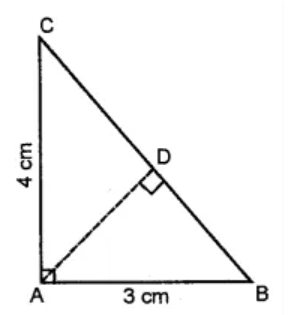# ML Aggarwal Solutions Class 8 Mathematics Solutions for Mensuration Exercise 18.1 in Chapter 18 - Mensuration

A copper wire when bent in the form of a square encloses an area of 121 cm2. If the same wire is bent into the form of a circle, find the area of the circle.

Given,

Area of the square = 121 cm2

So, side = √121 = 11 cm

Now,

Perimeter = 4 a = 4 × 11= 44 cm

And, the circumference of the circle = 44 cm

∴ Radius = (44 x 7)/ (2 x 22) = 7cm

Therefore, area of the circle = πr2 = (7)2

= 22/7 × 7 × 7= 154 cm2

Related Questions

Lido

Courses

Teachers

Book a Demo with us

Syllabus

Maths
CBSE
Maths
ICSE
Science
CBSE

Science
ICSE
English
CBSE
English
ICSE
Coding

Terms & Policies

Selina Question Bank

Maths
Physics
Biology

Allied Question Bank

Chemistry
Connect with us on social media!